# Nonlinear OpAmp Circuits

• #### Question 1

 Don’t just sit there! Build something!!

Learning to mathematically analyze circuits requires much study and practice. Typically, students practice by working through lots of sample problems and checking their answers against those provided by the textbook or the instructor. While this is good, there is a much better way.

You will learn much more by actually building and analyzing real circuits, letting your test equipment provide the “answers” instead of a book or another person. For successful circuit-building exercises, follow these steps:

1. Carefully measure and record all component values prior to circuit construction.
2. Draw the schematic diagram for the circuit to be analyzed.
3. Carefully build this circuit on a breadboard or other convenient medium.
4. Check the accuracy of the circuit’s construction, following each wire to each connection point, and verifying these elements one-by-one on the diagram.
5. Mathematically analyze the circuit, solving for all voltage and current values.
6. Carefully measure all voltages and currents, to verify the accuracy of your analysis.
7. If there are any substantial errors (greater than a few percent), carefully check your circuit’s construction against the diagram, then carefully re-calculate the values and re-measure.

Avoid using the model 741 op-amp, unless you want to challenge your circuit design skills. There are more versatile op-amp models commonly available for the beginner. I recommend the LM324 for DC and low-frequency AC circuits, and the TL082 for AC projects involving audio or higher frequencies.

As usual, avoid very high and very low resistor values, to avoid measurement errors caused by meter “loading”. I recommend resistor values between 1 kΩ and 100 kΩ.

One way you can save time and reduce the possibility of error is to begin with a very simple circuit and incrementally add components to increase its complexity after each analysis, rather than building a whole new circuit for each practice problem. Another time-saving technique is to re-use the same components in a variety of different circuit configurations. This way, you won’t have to measure any component’s value more than once.

• #### Question 2

We know that an opamp connected to a voltage divider with a voltage division ratio of $$\frac{1}{2}$$ will have an overall voltage gain of 2, and that the same circuit with a voltage division ratio of $$\frac{2}{3}$$ will have an overall voltage gain of 1.5, or $$\frac{3}{2}$$:There is definitely a mathematical pattern at work in these non-inverting opamp circuits: the overall voltage gain of the circuit is the mathematical inverse of the feedback network’s voltage gain.

Building on this concept, what do you think would be the overall function of the following opamp circuits?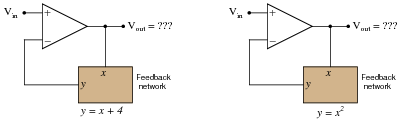• #### Question 3

The relationship between voltage and current for a PN junction is described by this equation, sometimes referred to as the “diode equation,” or “Shockley’s diode equation” after its discoverer:

 ID = IS (e[(qVD)/NkT] − 1)

Where,

ID = Current through the PN junction, in amps

IS = PN junction saturation current, in amps (typically 1 picoamp)

e = Euler’s number ≈ 2.718281828

q = Electron unit charge, 1.6 ×10−19 coulombs

VD = Voltage across the PN junction, in volts

N = Nonideality coefficient, or emission coefficient (typically between 1 and 2)

k = Boltzmann’s constant, 1.38 ×10−23

T = Junction temperature, degrees Kelvin

At first this equation may seem very daunting, until you realize that there are really only three variables in it: ID, VD, and T. All the other terms are constants. Since in most cases we assume temperature is fairly constant as well, we are really only dealing with two variables: diode current and diode voltage. Based on this realization, re-write the equation as a proportionality rather than an equality, showing how the two variables of diode current and voltage relate:

 ID ∝ . . .

Based on this simplified equation, what would an I/V graph for a PN junction look like? How does this graph compare against the I/V graph for a resistor?• #### Question 4

Plot the transfer function (Vout versus Vin) for this opamp circuit, and explain how the circuit works: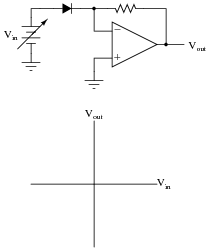What type of mathematical function is represented by this circuit?

• #### Question 5

Plot the transfer function (Vout versus Vin) for this opamp circuit, and explain how the circuit works:What type of mathematical function is represented by this circuit?

• #### Question 6

Plot the transfer function (Vout versus Vin) for this opamp circuit: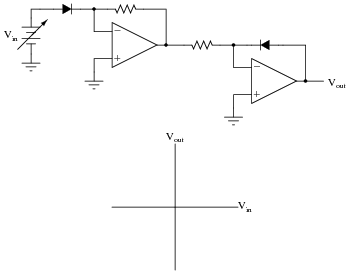What type of mathematical function is represented by this circuit?

• #### Question 7

Identify the mathematical function of this circuit (if you look closely, you’ll notice that the transistors are connected in such a way that they act very similar to diodes):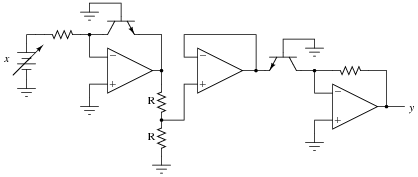Note: the two resistors labeled “R” are equal in value.

• #### Question 8

Identify the mathematical function of this circuit (if you look closely, you’ll notice that the transistors are connected in such a way that they act very similar to diodes):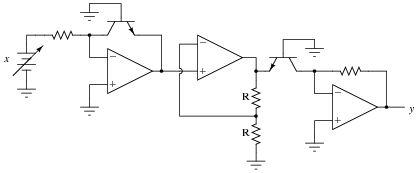Note: the two resistors labeled “R” are equal in value.

• #### Question 9

Suppose that in the course of building this exponential circuit you encounter severe inaccuracies: the circuit seems to work some of the time, but often its output deviates substantially (as much as /- 10%) from what it ought to be:Based on what you know of the components in this circuit, what could be varying so much as to cause these errors? What do you recommend as a solution to the problem?

• #### Question 10

Design an op-amp circuit that divides one quantity (x) by another quantity (y) using logarithms. To give you a start on this circuit, I’ll provide the initial logarithmic op-amp modules in this diagram: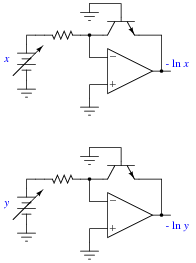Note: it will be helpful for your analysis to write the mathematical expression at each op-amp output in your circuit, so you may readily see how the overall math function is constructed from individual steps.

• #### Question 11

Find a datasheet for the AD538, an integrated circuit manufactured by Analog Devices. Then, read it carefully and explain how it is able to perform arithmetic functions such as multiplication, division, powers, and roots.

• #### Question 12

Predict how the operation of this exponentiator circuit will be affected as a result of the following faults. Consider each fault independently (i.e. one at a time, no multiple faults):Resistor R1 fails open:
Solder bridge (short) across resistor R1:
Diode D1 fails open:
Diode D1 fails shorted:

For each of these conditions, explain why the resulting effects will occur.

• #### Question 13

Predict how the operation of this logarithm extractor circuit will be affected as a result of the following faults. Consider each fault independently (i.e. one at a time, no multiple faults):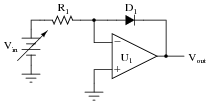Resistor R1 fails open:
Solder bridge (short) across resistor R1:
Diode D1 fails open:
Diode D1 fails shorted:

For each of these conditions, explain why the resulting effects will occur.

• #### Question 14

Explain why carefully matched resistors and transistors are necessary in log/antilog circuits, using your own words. Also, explain why the operational amplifiers themselves need not be matched as precisely as the discrete components.

• #### Question 15

This square root extraction circuit used to work fine, but then one day it stopped outputting the square root of the input signal, and instead simply reproduced the input signal with a gain of 1: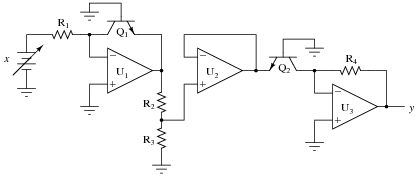What could have gone wrong with this circuit to cause it to stop “calculating” the square root of the input? Be as detailed as possible in your answer.

• #### Question 16

Identify at least two independent component faults which could cause this square root extraction circuit to always output 0 volts instead of the square root of input voltage x as it should:Explain why each of your proposed faults would cause the output to stay at 0 volts.

• #### Question 17

In the early 1970’s, the Fluke company invented a revolutionary new “RMS sensor” integrated circuit, used to convert an arbitrary waveform into its DC-equivalent (RMS) voltage. The device uses two precision resistors to heat a pair of matched transistors connected as a differential pair: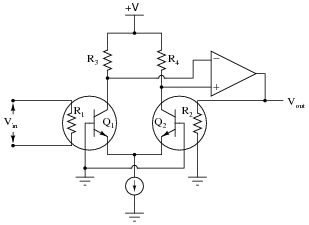Describe how this circuit functions. What physical principle(s) does it use to derive an RMS value for Vin? Why is it important that all identical components (transistors, resistors) be precisely matched?